# iBreakDown plots for classification models

#### 2020-04-20

Here we will use the HR data from DALEX package to present the iBreakDown for classification models.

# devtools::install_github("ModelOriented/DALEX")
library("DALEX")
library("iBreakDown")

head(HR)
#>   gender      age    hours evaluation salary   status
#> 1   male 32.58267 41.88626          3      1    fired
#> 2 female 41.21104 36.34339          2      5    fired
#> 3   male 37.70516 36.81718          3      0    fired
#> 4 female 30.06051 38.96032          3      2    fired
#> 5   male 21.10283 62.15464          5      3 promoted
#> 6   male 40.11812 69.53973          2      0    fired
new_observation <- HR_test[1,]
new_observation
#>   gender      age    hours evaluation salary status
#> 1   male 57.72683 42.31527          2      2  fired

## glm

First, we fit a model.

library("nnet")
m_glm <- multinom(status ~ . , data = HR, probabilities = TRUE, model = TRUE)
#> # weights:  21 (12 variable)
#> initial  value 8620.810629
#> iter  10 value 7002.127738
#> iter  20 value 6239.478146
#> iter  20 value 6239.478126
#> iter  20 value 6239.478124
#> final  value 6239.478124
#> converged

To understand the factors that drive predictions for a single observation we use the iBreakDown package.

However, sometimes we need to create custom predict function which returns probalilities.

p_fun <- function(object, newdata) {
if (nrow(newdata) == 1) {
as.matrix(t(predict(object, newdata, type = "prob")))
} else {
as.matrix(predict(object, newdata=newdata, type = "prob"))
}
}

Now we create an object of the break_down class. If we want to plot distributions of partial predictions, set keep_distributions = TRUE.

bd_glm <- local_attributions(m_glm,
data = HR_test,
new_observation =  new_observation,
keep_distributions = TRUE,
predict_function = p_fun)

We can simply print the result.

bd_glm
#>                                   contribution
#> multinom.fired: intercept                0.361
#> multinom.fired: evaluation = 2           0.084
#> multinom.fired: hours = 42.32            0.129
#> multinom.fired: gender = male           -0.007
#> multinom.fired: age = 57.73             -0.005
#> multinom.fired: salary = 2               0.002
#> multinom.fired: status = fired           0.000
#> multinom.fired: prediction               0.563
#> multinom.ok: intercept                   0.281
#> multinom.ok: evaluation = 2              0.134
#> multinom.ok: hours = 42.32              -0.016
#> multinom.ok: gender = male               0.006
#> multinom.ok: age = 57.73                 0.004
#> multinom.ok: salary = 2                 -0.002
#> multinom.ok: status = fired              0.000
#> multinom.ok: prediction                  0.407
#> multinom.promoted: intercept             0.358
#> multinom.promoted: evaluation = 2       -0.218
#> multinom.promoted: hours = 42.32        -0.113
#> multinom.promoted: gender = male         0.001
#> multinom.promoted: age = 57.73           0.002
#> multinom.promoted: salary = 2            0.000
#> multinom.promoted: status = fired        0.000
#> multinom.promoted: prediction            0.030

Or plot it.

plot(bd_glm)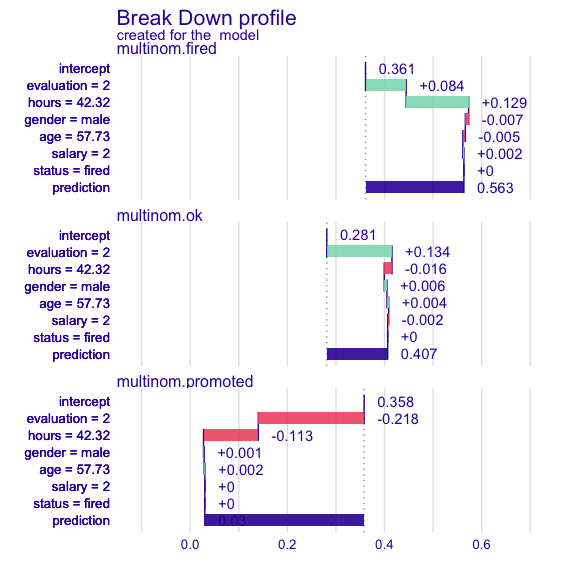Use the baseline argument to set the origin of plots.

plot(bd_glm, baseline = 0)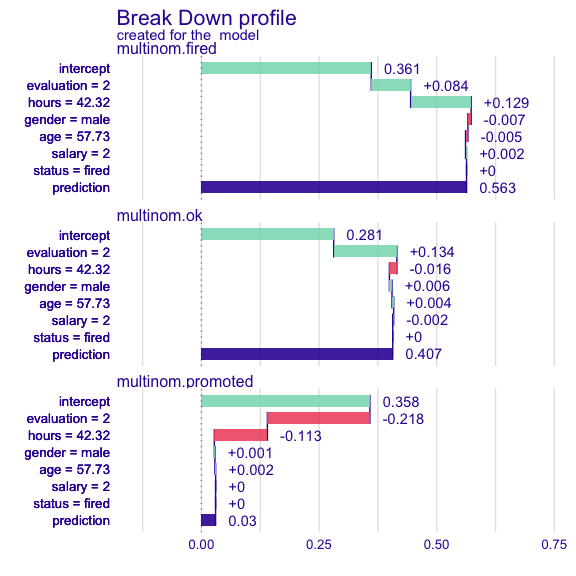Use the plot_distributions argument to see distributions of partial predictions.

plot(bd_glm, plot_distributions = TRUE)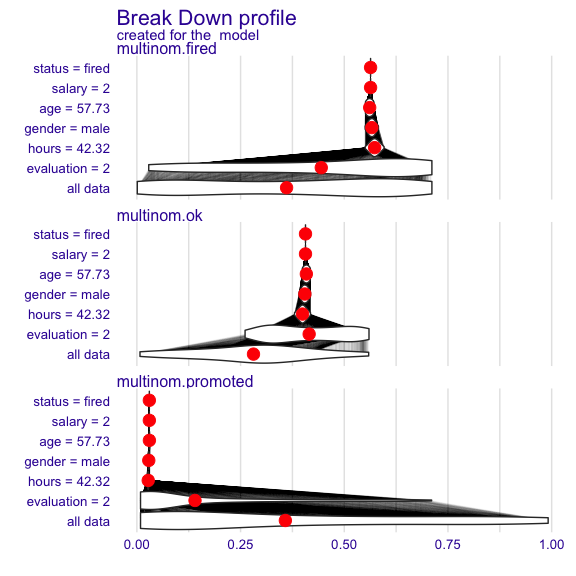For another types of models we proceed analogously.

## randomForest

library(randomForest)

m_rf <- randomForest(status ~ . , data = HR)

p_fun <- function(object, newdata){predict(object, newdata = newdata, type = "prob")}

data = HR_test,
new_observation =  new_observation,
predict_function = p_fun)

bd_rf
#>                                               contribution
#> randomForest.formula.fired: intercept                0.377
#> randomForest.formula.fired: hours = 42.32            0.234
#> randomForest.formula.fired: evaluation = 2           0.062
#> randomForest.formula.fired: salary = 2              -0.247
#> randomForest.formula.fired: gender = male            0.036
#> randomForest.formula.fired: age = 57.73              0.335
#> randomForest.formula.fired: status = fired           0.000
#> randomForest.formula.fired: prediction               0.796
#> randomForest.formula.ok: intercept                   0.270
#> randomForest.formula.ok: hours = 42.32              -0.046
#> randomForest.formula.ok: evaluation = 2              0.097
#> randomForest.formula.ok: salary = 2                  0.245
#> randomForest.formula.ok: gender = male              -0.039
#> randomForest.formula.ok: age = 57.73                -0.329
#> randomForest.formula.ok: status = fired              0.000
#> randomForest.formula.ok: prediction                  0.198
#> randomForest.formula.promoted: intercept             0.353
#> randomForest.formula.promoted: hours = 42.32        -0.188
#> randomForest.formula.promoted: evaluation = 2       -0.158
#> randomForest.formula.promoted: salary = 2            0.002
#> randomForest.formula.promoted: gender = male         0.003
#> randomForest.formula.promoted: age = 57.73          -0.005
#> randomForest.formula.promoted: status = fired        0.000
#> randomForest.formula.promoted: prediction            0.006
plot(bd_rf)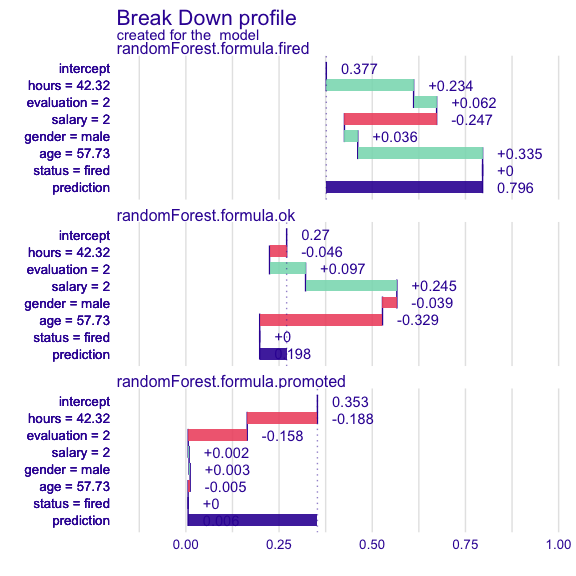plotD3(bd_rf)

## SVM

library(e1071)

m_svm <- svm(status ~ . , data = HR, type = "C-classification", probability = TRUE)

p_fun <- function(object, newdata){
p <- predict(object, newdata = newdata, probability = TRUE)
attr(p, "probabilities")
}

plot(bd_svm)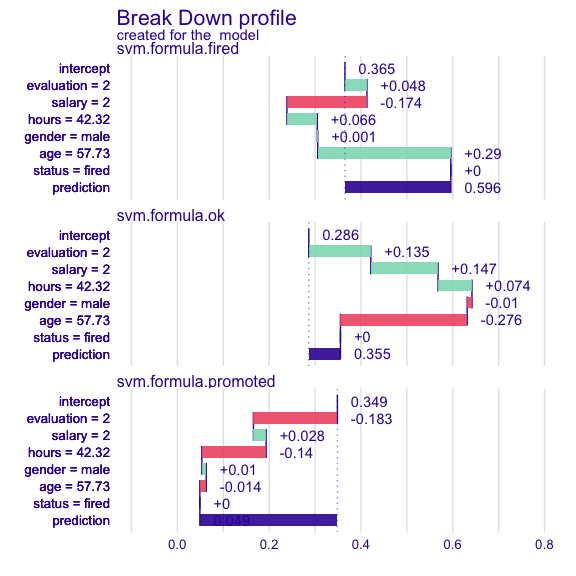plotD3(bd_svm)